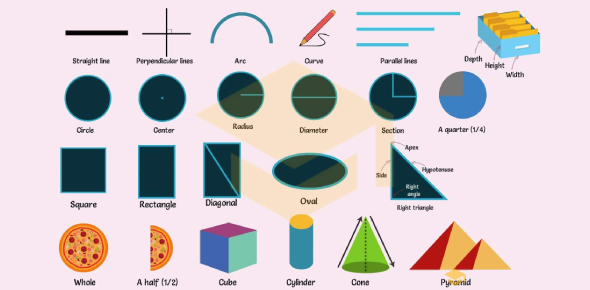# Statistics Concepts And Vocabulary Quiz! Trivia

10 Questions | Total Attempts: 51Settings.

• 1.
Asking the first ten people who walk by a question is an example of a..... ("And You Believed That?!")
• A.

Simple Random Sample (SRS)

• B.

Cluster Sample

• C.

Convenience Sampling

• D.

Voluntary Response

• E.

Systematic Sample

• 2.
Which of the following characteristics must a situation have to be considered binomial? ("One Way or the Other")
• A.

Each observation must have only two possible outcomes, either a success or a failure.

• B.

The observations/trials are dependent.

• C.

The probability of success is the same for each trial.

• D.

All of the above

• E.

Both A and C

• 3.
This bar graph showing the probability distribution of test scores is a....   ("Please Be Discrete" and "One Way or the Other")
• A.

Probability Histogram

• B.

Frequency Table

• C.

Binomial Distribution

• D.

Chance Graph

• E.

Pie Chart

• 4.
All are true of the Empirical Rule for Normal Distributions except that..... ("Let's Be Normal")
• A.

68% of the data falls within one standard deviation of the mean

• B.

It describes an asymmetrical bell-shaped curve.

• C.

95% of the data falls within two standard deviations of the mean

• D.

It is also referred to as the Three-Sigma Rule.

• E.

99.7% of the data falls within three standard deviations of the mean.

• 5.
All three bell curves pictured above share the same what?("Let's Be Normal")
• A.

Standard Deviation

• B.

Treatments

• C.

Mean

• D.

Z-Score

• E.

Focus of Study

• 6.
What is the name of the value indicating how many standard deviations a piece of data is from the mean? ("Let's Be Normal")
• A.

Z-Score

• B.

Distribution Range

• C.

Standard Data Value

• D.

Standard Normal Distribution

• E.

Long Range Variation

• 7.
Radio listeners calling in to answer a question is an example of a(n)... ("And You Believed That?!")
• A.

Explanatory Variable

• B.

Systematic Study

• C.

Voluntary Response

• D.

Convenience Sampling

• E.

Observational Study

• 8.
Binompdf is a measure of.....("One Way or the Other")
• A.

The probability that an exact number of successes will take place in a situation/trial

• B.

The probability that at least a certain number of successes will take place in a situation/trial

• 9.
Which of the following is not a key step in defining an observational study? ("We're Watching You")
• A.

Collecting the data

• B.

Determining the focus of the study

• C.

Asking around for instruments and necessary resources

• D.

Developing a plan to collect data

• E.

Reporting the findings of the study

• 10.
Which is not a basic principle of experimental design? ("We're Watching You")
• A.

Randomization

• B.

Grouping

• C.

Control

• D.

Replication

Related TopicsBack to top